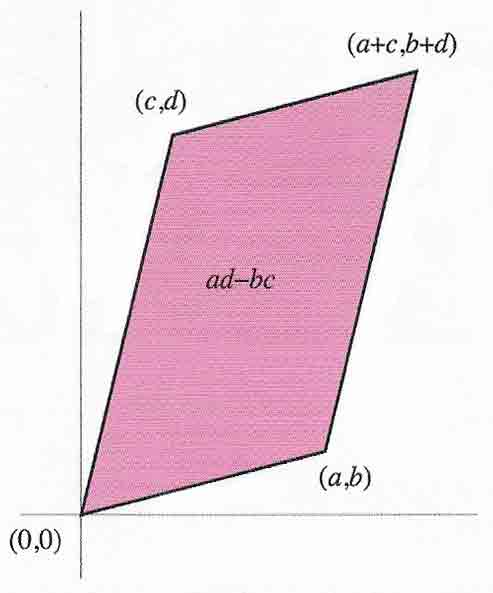A# vector spaceA quadrilateral formed by two vectors (a, b) and (c, d). Vectors may be added together and multiplied (scaled) by numbers called scalars.

A vector space, also known as a linear space, is the most fundamental concept in linear algebra. A vector space is a set of vectors together with a field of scalars such that: the sum of any two members of the set is a vector also in the set, and multiplication of a member of the set by a member of the field produces a vector also in the set. It is a generalization of the set of all geometrical vectors and is used throughout modern mathematics. Like the concepts of a group, a ring, and a field, that of a vector space is entirely abstract.

Italian mathematician Guiseppe Peano, a founder of mathematical logic and set theory, defined the vector space in his work Geometrical Calculation According to the Ausdehnungslhere of H. Grassmann, published in 1888. Mathematically a set of vectors form a vector space provided that it meets several conditions, such as closure under addition, the associative law, the commutative law, and the distributive law.

As an abstract way of extending Euclidean geometry's application to physical space, the vector space permits the scientist to assign numbers to given states of things at a certain time, and to calculate the results of forces acting on those things into the future. For example, suppose the things are physical particles when multiple forces simultaneously act on a particle at a point, each represented by a vector, the resulting combined force is easily calculated because forces combine as vectors add. A collection of points forming a field with infinite degrees of freedom can be acted upon by numerous simultaneous forces, and the subsequent behaviour of the field can be predicted.

After calculus, no mathematical technique is more useful across science than vector spaces and the linear algebra used with them. Modern theoretical physics — from classical mechanics, fluid theory, electromagnetic fiel theory, solid state physics, and particle physics, all the way to special and general relativity and quantum physics — relies extensively on them. English theoretical physics Paul Dirac found vector spaces crucial for handling the principle of superposition and other problems in quantum mechanics. Dirac said: "Now any mathematical quantities which can be added to give new quantities of the same nature may be represented by vectors in a suitable vector space with a sufficiently large number of dimensions". Even disciplines such as neuroscience make use of vector spaces.